Function Repository Resource:

# RegularHyperbolicTilingGraph

Create a graph of a regular hyperbolic tiling

Contributed by: Wolfram Physics Project Team
 ResourceFunction["RegularHyperbolicTilingGraph"][n,m,k] generates a graph corresponding to a tiling of the hyperbolic plane where m regular n-gons share a vertex, propagated for k steps.

## Details and Options

ResourceFunction["RegularHyperbolicTilingGraph"] gives an {n,m} regular hyperbolic tiling.
ResourceFunction["RegularHyperbolicTilingGraph"] is left unevaluated if the tiling specification is invalid.
ResourceFunction["RegularHyperbolicTilingGraph"] takes the same options as Graph.
Possible additional settings for the option GraphLayout include:
 "Beltrami" embed graph on the Beltrami-Klein disk "HalfPlane" embed graph on the Poincaré half-plane "Hemisphere" embed graph on a hemisphere (stereographic projection of Poincaré disk) "Hyperboloid" embed graph in the hyperboloid (Minkowski) model "Poincare" embed graph on the Poincaré disk

## Examples

### Basic Examples (2)

Graph of a {5,4} regular hyperbolic tiling:

 In:=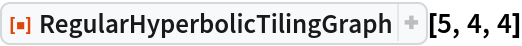Out=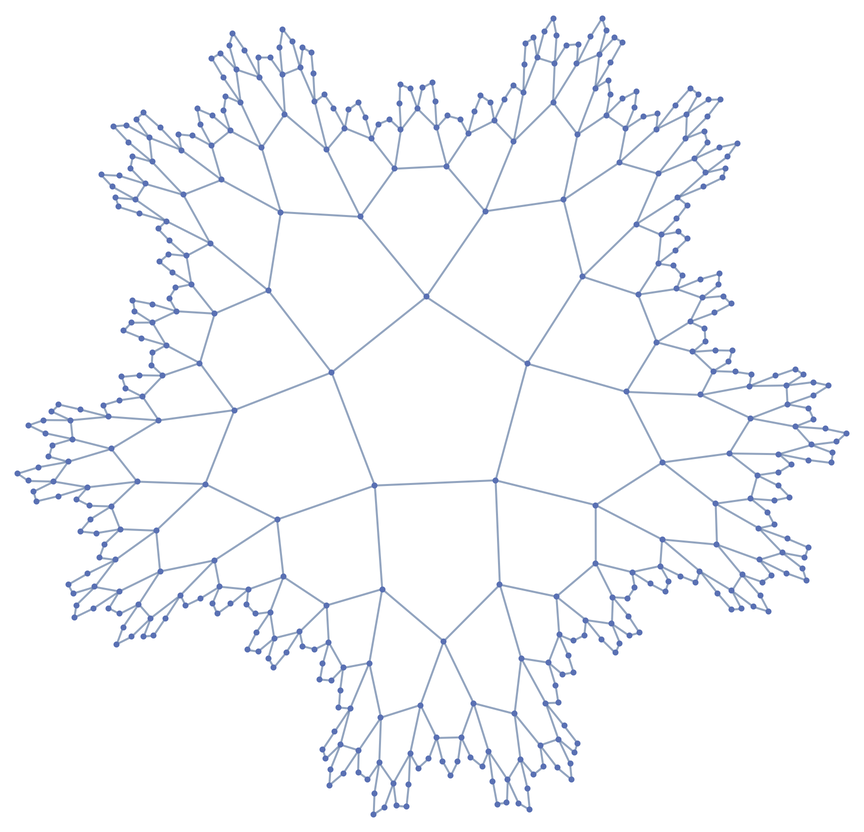Show the graph in 3D:

 In:=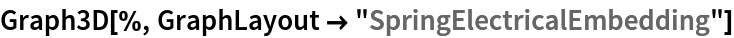Out=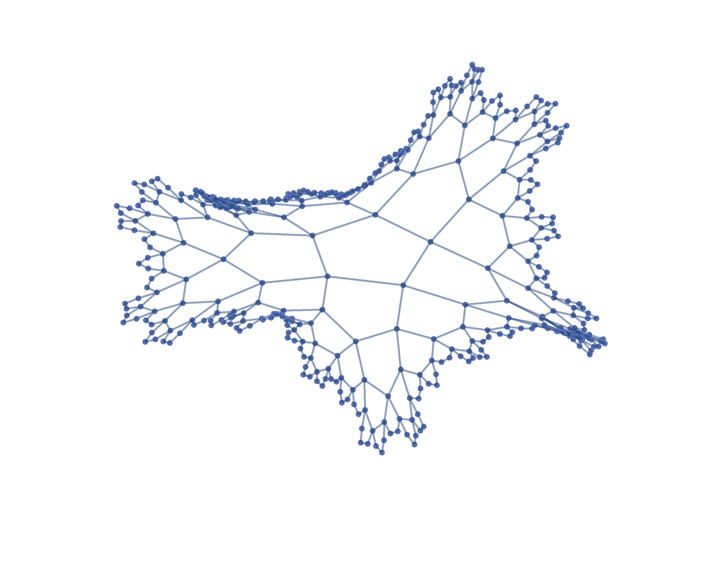### Scope (1)

Show the steps in generating a {6,4} regular hyperbolic tiling, embedded on the Poincaré disk:

 In:=Out=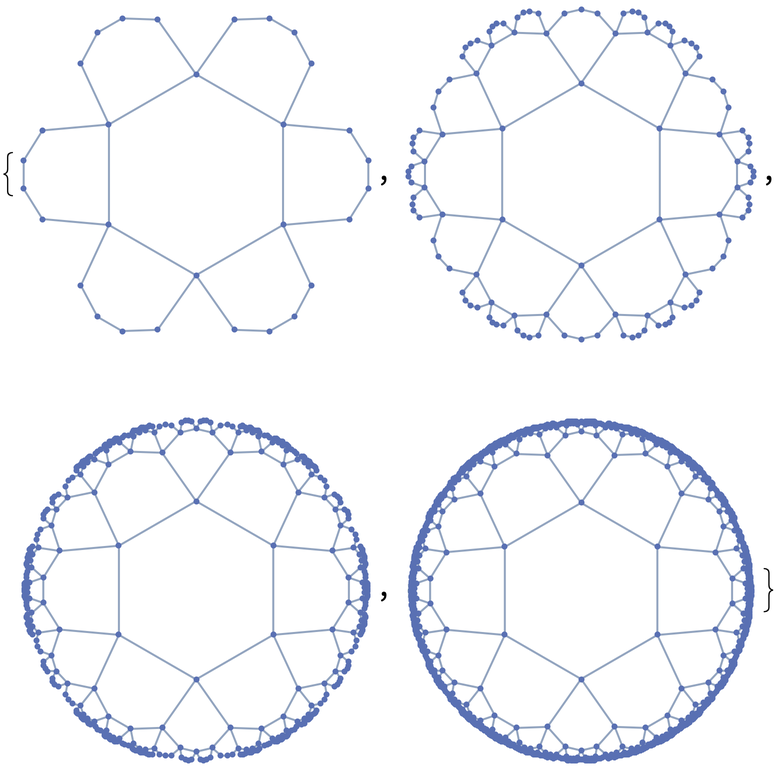### Options (6)

#### GraphLayout (6)

Embed the graph in the Beltrami-Klein disk:

 In:=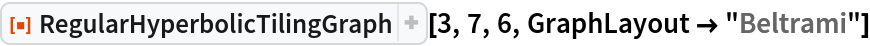Out=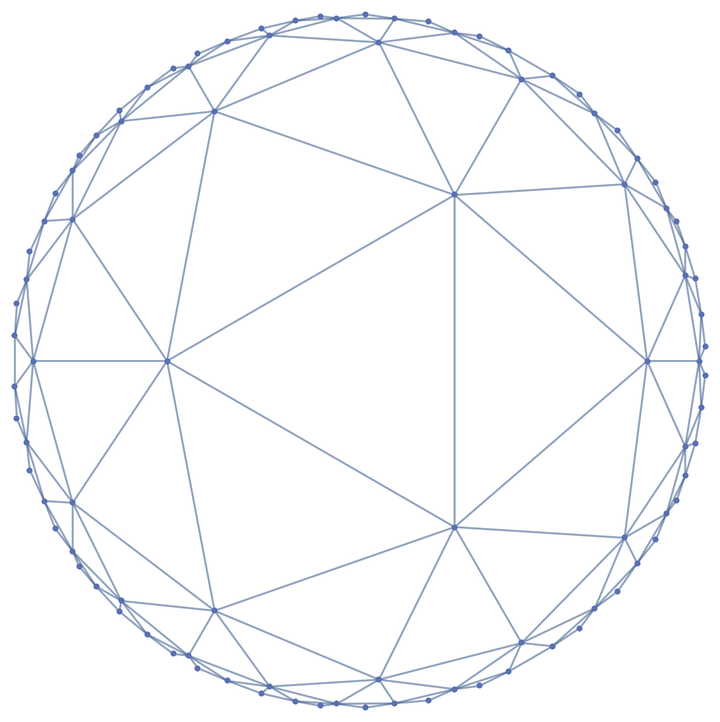Embed the graph in the Poincaré disk:

 In:=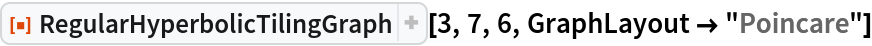Out=Embed the graph in the Poincaré half plane:

 In:=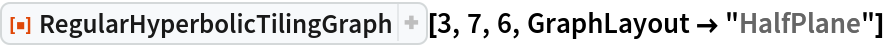Out=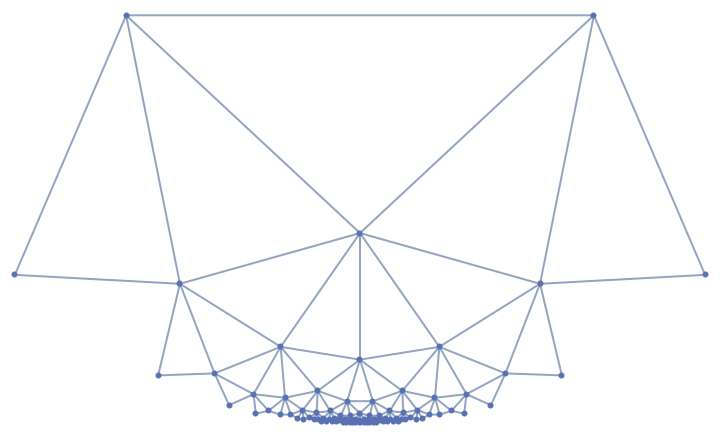Embed the graph in the hemisphere:

 In:=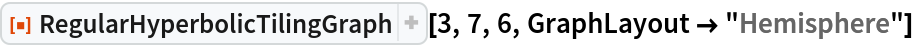Out=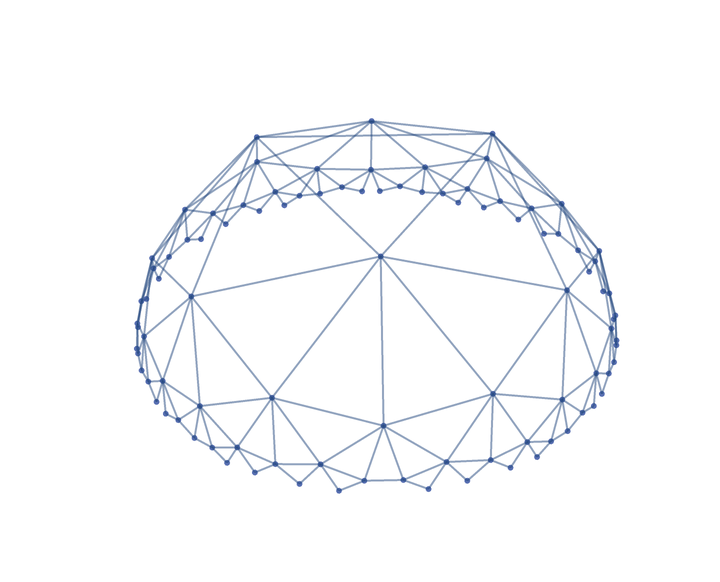Embed the graph in a hyperboloid:

 In:=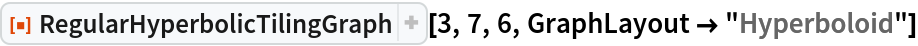Out=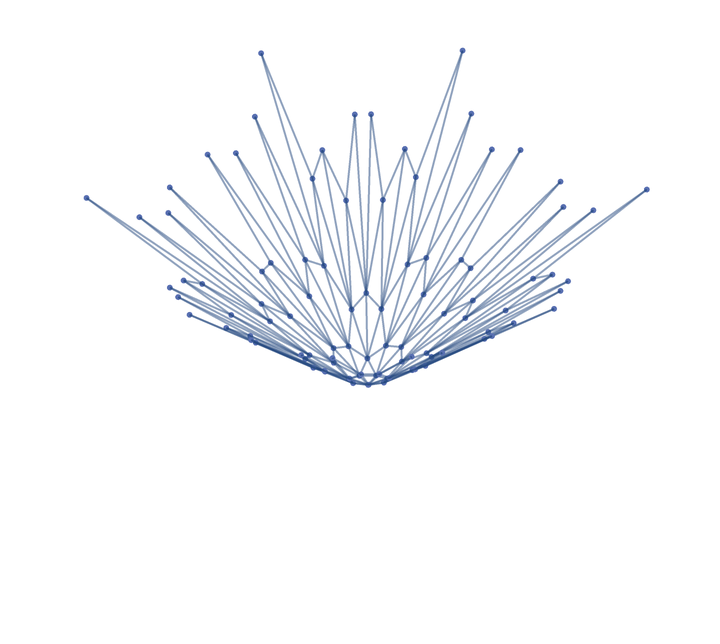Use an embedding supported by Graph:

 In:=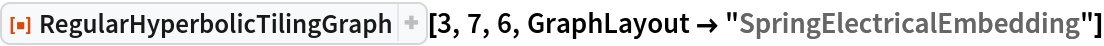Out=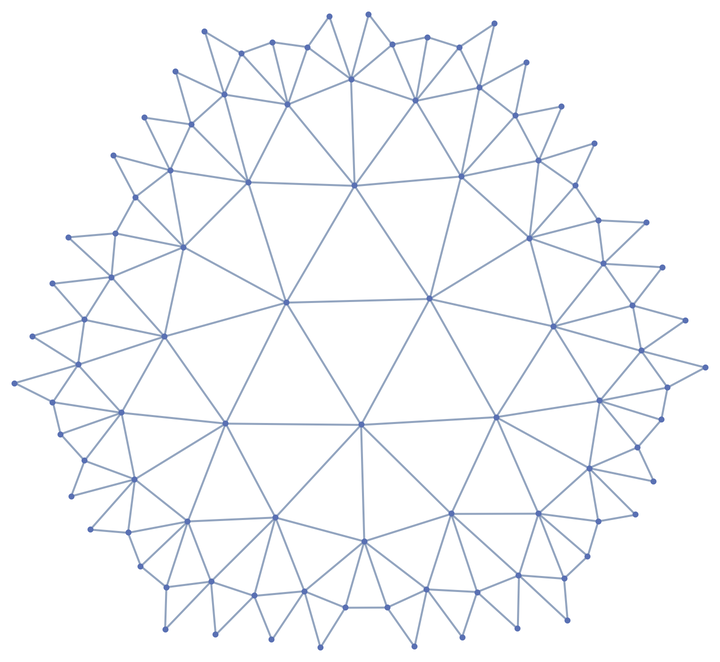### Possible Issues (2)

RegularHyperbolicTilingGraph returns unevaluated if the arguments do not correspond to a valid regular hyperbolic tiling:

 In:=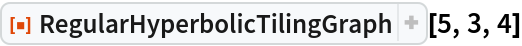Out=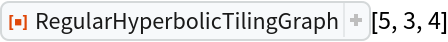For moderately sized arguments, generating the graph might take a long time:

 In:=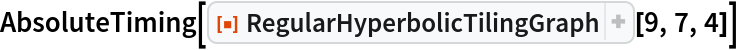Out=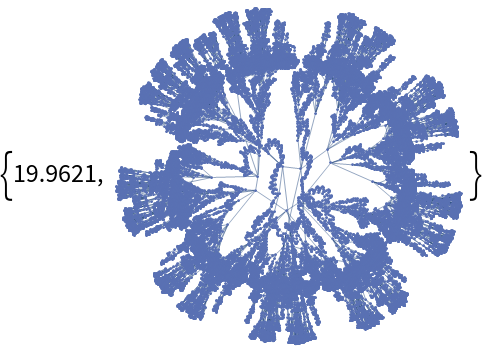## Version History

• 1.0.0 – 14 April 2020